# A Deep Learning Based Hybrid Approach for COVID-19 Disease Detections

A Deep Learning Based Hybrid Approach for COVID-19 Disease Detections
Computer Engineering Department, Fırat University, Elazig 23100, Turkey

Corresponding Author Email:
171129205@firat.edu.tr
Page:
461-468
|
DOI:
https://doi.org/10.18280/ts.370313
19 March 2020
|
Accepted:
1 May 2020
|
Published:
30 June 2020
| Citation

OPEN ACCESS

Abstract:

COVID-19 appeared in December 19, 2019 in Wuhan, China. This disease has spread to almost all countries in a short time. Countries take a series of stringent measures, including the prohibition of going out to prevent the virus that spreads COVID-19 disease. In this paper, we aimed to diagnose COVID-19 disease from X_RAY images by using deep learning architectures. In addition, 96.30% accuracy rate has been achieved with the hybrid architecture we have improved. While developing the hybrid model, the last 5 layers of Resnet 50 architecture were ejected. 10 layers were added in place of the 5 layers that were removed. The count of layers, which is 177 in the Resnet50 architecture, has been increased to 182 in the hybrid model. Thanks to these layer changes made in Resnet50, the accuracy rate has been increased more. Classification was performed with AlexNet, Resnet50, GoogLeNet, VGG16 and developed hybrid architectures using COVID-19 Chest X-Ray dataset and Chest X-Ray images (Pneumonia) datasets. As a result, when other scientific works in the literature are examined, it is finalized that the improved hybrid method offers better results than other deep learning architectures and can be used in computer-aided systems to diagnose COVID-19 disease.

Keywords:

Covid-19, deep learning, image processing, Resnet50, hybrid model

1. Introduction

The new corona virus (COVID-19) disease that has emerged in Wuhan, which is located in Hubei province of China, has no known vaccine and no effective treatment method . Rapid kits and PCR kits are available for diagnosis of the disease. Fast kits have lower accuracy than PCR kits. Experts are trying to develop vaccines for the treatment of the disease . It is said that even if the vaccine is developed according to the best situation, all tests and market launch may take up to a year . The COVID-19 virus has spread to many countries as of now. The number of cases and the number of people who lost their lives is quite high . Early diagnosis of COVID-19 disease and quarantine of the infected patient are vital for the massive spread of the disease and to combat the disease .

In this paper, Resnet50 architecture was used as a basis. A new hybrid model was proposed by improving the Resnet50 architecture. Thanks to the developed Hybrid model, an accuracy of 96.30% was achieved. this accuracy rate is one of the highest accuracy rates in the literature.

The clinical symptoms of COVID-19 disease in the patient are sudden fever, cough, shortness of breath and respiratory distress . However, these symptoms are not specific. It was determined that pneumonia was detected in chest CT scan in asymptomatically infected patients and that the virus was positive as a result of pathogenic testing. Radiological imaging is a very important diagnostic tool for the diagnosis of COVID-19 due to such situations .

More than 100 scientific articles have been published in the literature about COVID-19 disease in a very short time. Among these studies, the classification of the chest images by computer and the ones related to machine learning methods are as follows;

In their study, Wang et al. Applied 217 images of 453 CT images of patients confirmed to be COVID-19 in the CNN algorithm for the training of the system. They achieved a success rate of 83% accuracy .

In their study, Xu et al. Segmented candidate infection sites from the CT image set using a 3D deep learning model. They categorized these segments into segments using COVID-19, Influenza-A and unrelated groups of infections using the position-attention classification model with their corresponding confidence values. The data set they use contains 618 CT images. These images were performed by taking 219 CT samples from 110 COVID-19 patients, 224 influenza A samples and 175 CT samples from healthy people. In their method, they classified COVID-19 disease with an accuracy of 86.7% .

Wang and his colleagues proposed a new and effective Respiratory Simulation Model (RSM) in their work. With this model, 6 important clinical respiratory samples were classified. They have reached 94.5% accuracy rate with the system they have proposed .

In their study, Rao et al. Suggested that the possible case definitions of COVID-19 can be determined more quickly with a mobile phone-based web survey using machine learning algorithms. They also stated that this would reduce the speed of propagation, which is sensitive .

Shan and colleagues used DL-based segmentation in their studies and used the "VB-Net" neural network to segment COVID-19 infected areas in CT scans. They used 219 COVID-19 data. They stated that it showed high accuracy for quantitative evaluation, automatic infection site identification and POI measurements .

In their study, Gozes and colleagues have tried to develop artificial intelligence-based automated computerized imaging (CT) image analysis tools to detect, quantify and monitor COVID-19 and to show that they can distinguish COVID-19 patients from those without the disease. 157 patients in China and America were used in test studies. They stated that, using standard machine learning techniques and innovative artificial intelligence applications, together with a built-in computed tomography (CT) detection platform, it can be used as an effective tool for screening and early detection of patients who may have caught the COVID-19 pathogen .

In the continuation of the paper, material and methods, Application and Results, Conclusion sections are examined.

2. Material and Methods

In this article, it is aimed to classify diseases with deep learning architectures using COVID-19 Chest X-Ray dataset and Chest X-Ray images (Pneumonia) datasets. Deep learning architectures, which are a sub-branch of machine learning, have become very popular recently and these architectures are widely used. In this study, CNN architectures, a sub-branch of deep learning, were used. The developed model has been compared with CNN architectures. The data sets used, the structure of the developed method and the layers used are examined in the following section.

2.1 Dataset

The COVID-19 Chest X-Ray dataset and Chest X-Ray images (Pneumonia) datasets used in this study were taken from the Kaggle website, which is open access. The COVID-19 Chest X-Ray dataset used consists of 136 data in total. 245 normal images, 162 pneumonia data of Chest X-Ray images (Pneumonia) data set were used . The image samples used in the datasets are given in Figure 1.

Dataset

COVID-19

Pneumonia

Normal

## 1a.png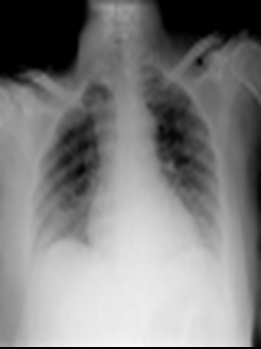## 1b.png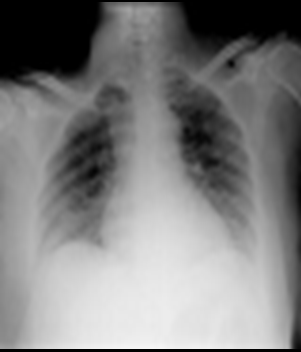## 1c.png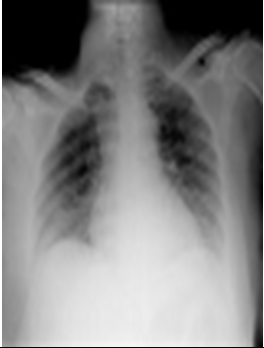Number of Data

136

162

245

Figure 1. Image examples used

2.2 Structure of systems

In the hybrid method, Resnet50 architecture was used as the basement. The input layer of the Resnet50 architecture has been updated to 224 * 224 * 1. Later, the Convolution layer after the input layer was replaced. Finally, the five layers of the Resnet50 architecture were extracted and ten new layers were added instead.

Thanks to the new layers added, the accuracy rate of the resnet50 model has been increased. Resnet50 architecture has been used as the basis in the developed hybrid model, as it achieves high performance in biomedical images.

The reason for choosing the Resnet50 architecture is that instead of training a network from scratch, a trained network will be more efficient. The existing knowledge of this model has been used. After the changes made in the Resnet50 architecture, the number of layers increased from 177 to 182 . The improved model is presented in Figure 2.

## 2.jpg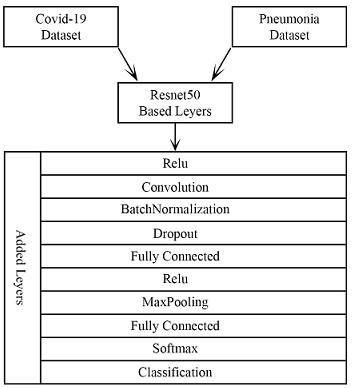Figure 2. Architecture of the hybrid model

Added layers, parameter numbers and other features are presented in Table 1.

Table 1. Properties of layers used in hybrid model

 Name Type Activations 1 Imageinput Image Input 224x224x1 2 conv_1 Convolution 112x112x64 172 add_16 Addition 7x7x2048 173 relu Relu 7x7x2048 174 conv_2 Convolution 7x7x32 175 batchnorm Batch Normalization 7x7x32 176 dropout Dropout 7x7x32 177 fc_1 Fully Connected 1x1x2 178 activation Relu 1x1x2 179 maxpool Max Pooling 1x1x2 180 fc_2 Fully Connected 1x1x2 181 fc1000_soft Softmax 1x1x2 182 classoutput Classification Output -
2.2.1 Input layer

This layer is the first layer of the developed hybrid model and other models. The images are first read from the input layer . The input sizes of the hybrid model and other models used in the application are in Table 2.

Table 2. Input size of images

 Model Input Size of Image Hybrid Model 224 224 3 GoogLeNet 224 224 3 AlexNet 227 227 3 Densenet201 224 224 3 InceptionV3 299 299 3 Resnet50 224 224 3
2.2.2 Convolutional layer

In this layer, the input image is reduced to a smaller size than the size of the filter used. NxN size filters can be preferred in this layer. The aim of this layer can be expressed shortly as producing feature maps . The discrete time convolution process is presented in Eq. (1).

$s(t)=(x * w)(t)=\sum_{a=-\infty}^{\infty} x(a) w(t-a)$  (1)

w: kernel (filter), $x:$ input, $t:$ times, $s:$ Result $m, n: 0$

If two-dimensional data is taken as input value, Eq. (2) is preferred.

$S(i, j)=(I * K)(i, j)$$=\sum_{m} \sum_{n} I(i, j) K(i-m, j-n) (2) The terms i and j indicate the locations of the new matrix acquired after the convolution process. The preferred method in this process is positioned so that the center of the filter is at the starting point. If cross entropy is to be performed, Eq. (3) is used. S(i, j)=(I * K)(i, j)$$=\sum_{m} \sum_{n}(i+m, j+n) K(m, n)$  (3)

2.2.3 Activation function

Activation functions are often preferred in artificial neural networks for nonlinear transformation processes. There are many activation functions developed in the literature. Relu, Sigmoid and Tanh are the most preferred among these activation functions. Relu was preferred in the hybrid model we developed . Activation functions are frequently used in deep learning models. Relu, Sigmoid and Tanh activation functions are given in Eqns. (4), (5), (6).

Relu: $f(x)=\left\{\begin{array}{l}0, x<0 \\ x, x \geq 0\end{array}, f(x)^{\prime}=\left\{\begin{array}{l}0, x<0 \\ 1, x \geq 0\end{array}\right.\right.$  (4)

Sigmoid: $f(x)=\frac{1}{1+e^{-x}}, f^{\prime}(x)=f(x)(1-f(x))$ (5)

Tanh: $f(x)=\tanh (x)=\frac{2}{1+e^{-2 x}}-1, f^{\prime}(x)=1-f(x)^{2}$ (6)

2.2.4 Normalization

This layer is preferred to normalize the output value produced by the convolution and fully connected layers. This layer briefly normalizes the layer output . In this way, the training period of the network is shortened and the network performs the learning process more quickly. Eq. (7) is used to perform normalization.

$Y_{i}=\frac{X i-\mu_{\beta}}{\sqrt{\sigma_{\beta}^{2}+\epsilon}}$  (7)

$\sigma_{\beta}=\frac{1}{M} \sum_{i=1}^{M}\left(X_{i}-\mu_{\beta}\right)^{2}$ (8)

$\mu_{\beta}=\frac{1}{M} \sum_{i=1}^{M} X_{i}$ (9)

M: Number of input data

$X_i$: i=1 ... M

$\mu_\beta$: Average value of the stack

$\sigma_\beta$: Standard deviation of the stack

$Y_{i}$: New values resulting from normalization process

2.2.5 Dropout

The Dropout layer is used to prevent the network from memorizing. Model can memorize training data and perform over-learning. If the network goes into an extreme learning process, it loses its ability to learn. With the dropout process, some nodes in the network are randomly disabled . In this way, the network is prevented from memorizing. Dropout process cannot be used in test and confirmation steps. A general dropout process is shown in Figure 3.

## 3.jpg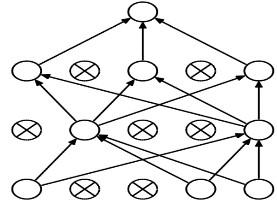Figure 3. Dropout process

2.2.6 Fully connected

The Fully Linked layer reduces the input data to a one-dimensional matrix format. The number of fully bound layers used in each architecture is different . Eq. (10) is used for this process.

$u_{i}^{l}=\sum_{j} w_{j i}^{l-1} y_{j}^{l-1}$  (10)

$y_{i}^{l}=f\left(u_{i}^{l}\right)+b^{(l)}$  (11)

l: Layer number,

i, j: Neuron number,

yli: the value in the output layer created,

wl-1ji: The weight value in the hidden layer,

yl-1i: The value of input neurous

uli: The value of the output layer

b(l): deviation value.

In this study, the number of classes (COVID-19, Pneumonia and Normal) is 3. For this reason, the output value of the fully connected layer 3 of our hybrid model is entered.

2.2.7 Pooling layer

This layer is a preferred layer after the convolution layer. With the pooling process, the information from the convolution layer is simplified. The most common pooling methods are average pooling and maximum pooling. In pooling, the network does not perform any learning. NxN sized filters are preferred for pooling process . The pooling process is given in Eq. (12). Maximum pooling is used in the developed hybrid model.

$S=w 2 * h 2 * d 2$  (12)

$w 2=\frac{(w 1-f)}{A+1}$  (13)

$h 2=\frac{h 1-f}{A+1}$  (14)

$d 2=d 1$  (15)

A = number of steps used

w1 = width of the input image,

h1 = height of the input image,

d1 = depth value of input image size,

f = filter size

S = Size of manufactured image.

Max-pooling was used in the hybrid architecture we developed.

2.2.8 SoftMax

It is accessed prior to the SoftMax classification layer . performs the probabilistic computation created on the network and generates a value for each class. The SoftMax process is given in Eq. (16).

$P(y=j \mid x ; W, b)=\frac{\exp ^{X^{T} W_{j}}}{\sum_{j=1}^{n} \exp ^{X^{T} W_{j}}}$  (16)

W, b, s a: weight vector.

2.2.9 Classification

This layer is the last layer of the architectures used to produce output value .

3. Application and Results

In this paper, COVID-19 x-ray chest images, and Chest X-Ray images (Pneumonia) images obtained before COVID-19 disease appeared, were combined. It is aimed to classify these combined data sets with deep learning architectures and the developed hybrid model. While 80% of datasets are used for education, 20% are used for testing. The application was obtained in a computer with an i5 processor, 8 GB RAM memory in Matlab environment .

One of the most important criteria in CNN architectures is the confusion matrix . Values such as Accuracy, Sensitivity, Specifity, F1 Score are calculated using the confusion matrix . In summary, the confusion matrix can be said to be the photo of the trained network. In general, a confusion matrix structure is presented in Table 3.

Table 3. Confusion matrix

 A B A TP FP B FN TN
TP(True-Positive): Data A was correctly predicted and placed in the correct class.

FP(False-Positive): Data A was estimated as B and was placed in the wrong class.

FN(False-Negative): The data B is estimated to be A but data is B.

TN(True-Negative): The data B is estimated to be B and data is actually B.

Accuracy: It is the proportion of the number of accurately estimated data to the total size of data used . The equation that calculates the accuracy value is given in Eq. (17).

Accuracy $=\frac{T P+T N}{T P+T N+F P+F N}$  (17)

The equation of the Sensitivity value obtained using the Confusion matrix is given in Eq. (18).

Sensitivity $(T P R)=\frac{T P}{T P+F N}$  (18)

The equation of the specificity value is presented in Eq. (19).

Specificity $(T N R)=\frac{T N}{T N+F P}$  (19)

Calculating F1 Score value is presented in Eq. (20), precision calculation in Eq. (21), Calculation of Recall value in Eq. (22), FPR in Eq. 23, FDR in Eq. (24) and FNR in Eq. (25).

$F-$measure$=\frac{2 * \text {Precision} * \text {Recall}}{\text {Precision}+\text {Recall}}$ (20)

Precision $=\frac{T P}{T P+F P}$ (21)

Recall $=\frac{T P}{T P+F N}$  (22)

$\text {False Positive Rate }(F P R)=\frac{F P}{F P+T N}$  (23)

$\text {False Discovery Rate }(F D R)=\frac{F P}{F P+T P}$  (24)

$\text {False Negative Rate }(F D R)=\frac{F N}{F N+T P}$   (25)

Cnn architectures and training data used in the developed model are given in Table 4.

Table 4. Educational values of models

 Solver Name Sgdm MaxEpochs 4 MiniBatchSize 10 Shuffle every-epoch ValidationFrequency 6 InitialLearnRate 1.000e-04 Total Iteration 172
The format of the confusion matrix presented in the application is as in Table 5.

Table 5. Confusion matrix in the application

 COVID-19 Pneumonia Normal COVID-19 True False False Pneumonia False True False Normal False False True
The accuracy and loss curves obtained with the improved hybrid model are shown in Figure 4.

## 4.jpg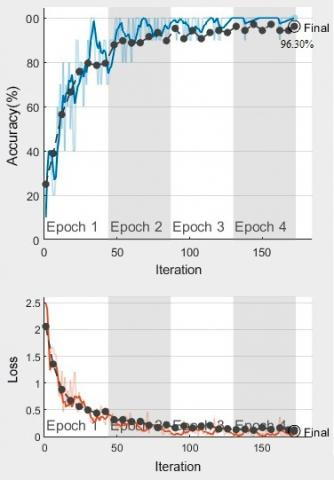Figure 4. Accuracy and loss curves of the improved model

## 5.jpg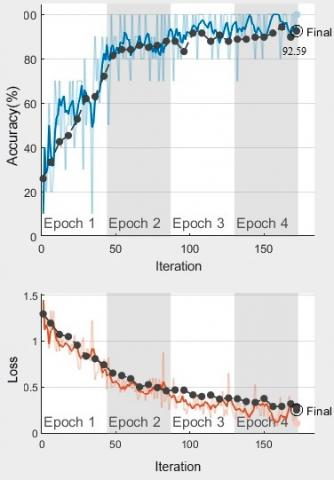Figure 5. Accuracy and loss curves of the Resnet50

After the network is trained, the performance values of the network are given in Table 6.

Accuracy, Sensitivity, Specificity, F1 Measure, FPR, FDR, FNR values were obtained by multiplying 100 in all architectures. These values were calculated separately for COVID-19, Pneumonia and Normal images class.

The accuracy and loss curves obtained with the Resnet50 model are shown in Figure 5.

After the network is trained, the performance values of the network are given in Table 7.

The accuracy and loss curves obtained with the AlexNet model are shown in Figure 6.

After the network is trained, the performance values of the network are given in Table 8.

Table 6. Performance value of the Improved model

 Confusion Matris 26 0 1 0 49 0 1 2 29 COVID-19 Pneumonia Normal Accuracy 98.11 98.11 96.30 Sensitivity 96.30 96.08 96.67 Specificity 98.73 1 96.5 F1 Score 96.30 98.00 93.55 FPR 1.27 0 3.85 FDR 3.70 0 9.38 FNR 3.70 3.92 3.33

Table 7. Performance value of the Resnet50

 Confusion Matris 26 0 1 0 48 1 2 4 26 COVID-19 Pneumonia Normal Accuracy 97.09 95.24 92.59 Sensitivity 92.86 92.31 92.86 Specificity 98.67 98.11 92.50 F1 Score 94.55 95.05 86.67 FPR 1.33 1.89 7.50 FDR 3.70 2.04 18.75 FNR 7.14 7.69 7.14

## 6.jpg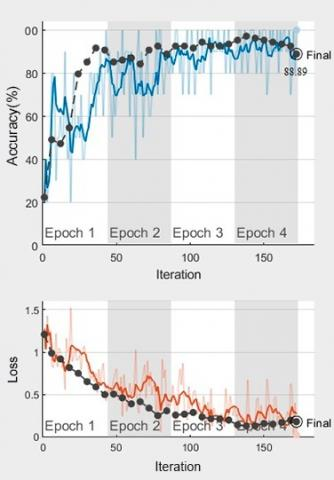Figure 6. Accuracy and loss curves of the AlexNet

Table 8. Performance value of the AlexNet

 Confusion Matris 27 0 0 0 48 1 5 6 21 COVID-19 Pneumonia Normal Accuracy 95.05 93.20 88.89 Sensitivity 84.38 88.89 95.45 Specificity 1 97.96 87.21 F1 Score 91.53 93.20 77.78 FPR 0 02.04 12.79 FDR 0 02.04 34.38 FNR 15.63 1.11 4.55
The accuracy and loss curves obtained with the GoogLeNet model are shown in Figure 7.

## 7.jpgFigure 7. Accuracy and loss curves of the GoogLeNet

After the network is trained, the performance values of the network are given in Table 9.

Table 9. Performance value of the Google net

 Confusion Matris 27 0 0 0 49 0 2 8 22 COVID-19 Pneumonia Normal Accuracy 98.00 92.45 90.74 Sensitivity 93.10 85.96 1 Specificity 1 1 88.37 F1 Score 96.43 92.45 81.48 FPR 0 0 11.63 FDR 0 0 31.25 FNR 6.90 14.04 0
The accuracy and loss curves obtained with the Vgg16 model are shown in Figure 8.

## 8.jpg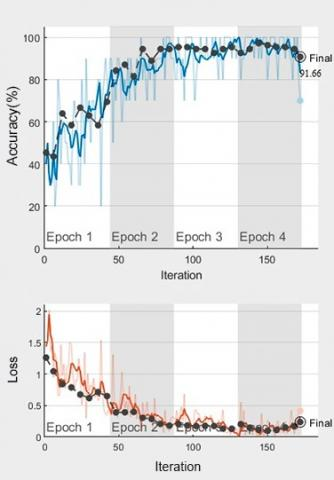Figure 8. Accuracy and loss curves of the Vgg16

After the network is trained, the performance values of the network are given in Table 10.

Table 10. Performance value of the Vgg16

 Confusion Matris 25 0 2 1 43 5 1 0 31 COVID-19 Pneumonia Normal Accuracy 96.12 94.29 92.52 Sensitivity 92.59 1 96.88 Specificity 97.37 90.32 90.67 F1 Score 92.59 93.48 88.57 FPR 2.63 9.68 9.33 FDR 7.41 12.24 18.42 FNR 7.41 0 3.13

## 9.png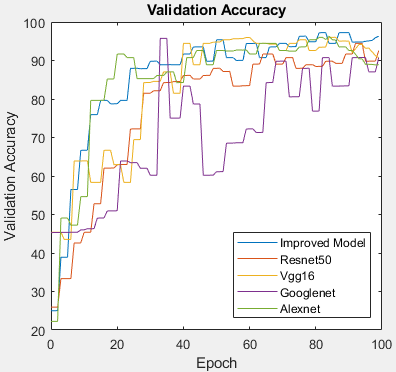Figure 9. Accuracy curves of models

## 10.jpg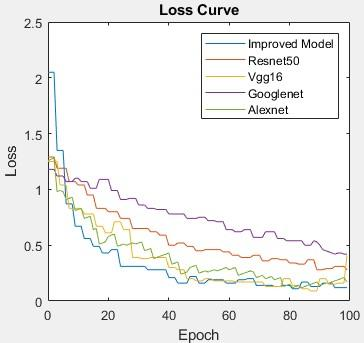Figure 10. Loss curves of models

Although the accuracy curves of the models used in this paper are shown in Figure 9, the loss curves are presented in Figure 10.

Accuracy value are given in Table 11 of all models used in the study.

The literature studies on COVID-19 are presented in Table 12.

Table 11. Accuracy value of all models

 Accuracy Hybrid Model 96.30 Resnet50 92.59 Vgg16 91.66 GoogLeNet 90.74 AlexNet 88.89

Table 12. Studies on COVID-19
 Authors/Year Methods Accuracy Wang et al.  /2020 CNN 83.00% Xu et al.  /2020 Deep Learning 86.7% Wang et al. /2020 Machine Learning - Rao et al.  Machine Learning - Shan et al.  /2020 Segmentation - Gozes et al.  /2020 artificial intelligence -
4. Conclusion

COVID-19 disease occurs in almost all countries of the world shortly after it appeared in China in December 2019. Countries take various measures to combat this disease, which has a high risk of transmission. The scientific world is spending an extensive time working on both the detection and treatment of the disease. In our study for the diagnosis of the disease, we tried to diagnose the disease using X-ray images. In this study, CNN architectures were used to diagnose COVID-19 disease. In this study, a hybrid model that we developed for the diagnosis of COVID-19 was used. In this developed model, Resnet50, one of the CNN architectures, was used as the base. By removing 5 layers of the Resnet50 model, 10 new layers were added to the Resnet50. With this developed hybrid model, an accuracy rate of 96.30% was achieved. At the same time, results were acquired with AlexNet, Resnet50, Vgg16 and GoogLeNet architectures. The highest accuracy rate was achieved with the hybrid model we improved.

References

 Zhou, F., Yu, T., Du, R., Fan, G., Liu, Y., Liu, Z., Guan, L. (2020). Clinical course and risk factors for mortality of adult inpatients with COVID-19 in Wuhan, China: A retrospective cohort study. The Lancet, 395: 1054-1062. https://doi.org/10.1016/S0140-6736(20)30566-3

 Rasmussen, S.A., Smulian, J.C., Lednicky, J.A., Wen, T.S., Jamieson, D.J. (2020). Coronavirus Disease 2019 (COVID-19) and pregnancy: What obstetricians need to know. American Journal of Obstetrics and Gynecology, 415-426. https://doi.org/10.1016/j.ajog.2020.02.017

 Qiu, H., Wu, J., Hong, L., Luo, Y., Song, Q., Chen, D. (2020). Clinical and epidemiological features of 36 children with coronavirus disease 2019 (COVID-19) in Zhejiang, China: An observational cohort study. The Lancet Infectious Diseases, 20: 689-696. https://doi.org/10.1016/S1473-3099(20)30198-5

 Remuzzi, A., Remuzzi, G. (2020). COVID-19 and Italy: What next? The Lancet, 395: 1225-1228. https://doi.org/10.1016/S0140-6736(20)30627-9

 Zhang, S., Diao, M., Yu, W., Pei, L., Lin, Z., Chen, D. (2020). Estimation of the reproductive number of novel coronavirus (COVID-19) and the probable outbreak size on the Diamond Princess cruise ship: A data-driven analysis. International Journal of Infectious Diseases, 93: 201-204. https://doi.org/10.1016/j.ijid.2020.02.033

 Wang, D., Hu, B., Hu, C., Zhu, F., Liu, X., Zhang, J. (2020). Clinical characteristics of 138 hospitalized patients with 2019 novel coronavirus-infected pneumonia in Wuhan, China. Jama, 323(11): 1061-1069. https://doi.org/10.1001/jama.2020.1585

 Chen, N., Zhou, M., Dong, X., Qu, J., Gong, F., Han, Y. (2020). Epidemiological and clinical characteristics of 99 cases of 2019 novel coronavirus pneumonia in Wuhan, China: A descriptive study. Lancet, 395: 507-513. https://doi.org/10.1016/S0140-6736(20)30211-7

 Wang, S., Kang, B., Ma, J., Zeng, X., Xiao, M., Guo, J., Xu, B. (2020). A deep learning algorithm using CT images to screen for Corona Virus Disease (COVID-19). medRxiv. https://doi.org/10.1101/2020.02.14.20023028

 Xu, X., Jiang, X., Ma, C., Du, P., Li, X., Lv, S., Li, Y. (2020). Deep learning system to screen coronavirus disease 2019 pneumonia. arXiv preprint arXiv:2002.09334.

 Wang, Y., Hu, M., Li, Q., Zhang, X.P., Zhai, G., Yao, N. (2020). Abnormal respiratory patterns classifier may contribute to large-scale screening of people infected with COVID-19 in an accurate and unobtrusive manner. arXiv preprint arXiv:2002.05534.

 Rao, A.S.S., Vazquez, J.A. (2020). Identification of COVID-19 can be quicker through artificial intelligence framework using a mobile phone-based survey in the populations when cities/towns are under quarantine. Infection Control & Hospital Epidemiology, 1-18. https://doi.org/10.1017/ice.2020.61

 Shan, F., Gao, Y., Wang, J., Shi, W., Shi, N., Han, M., Shi, Y. (2020). Lung infection quantification of COVID-19 in CT images with deep learning. arXiv preprint arXiv:2003.04655.

 Gozes, O., Frid-Adar, M., Greenspan, H., Browning, P.D., Zhang, H., Ji, W., Siegel, E. (2020). Rapid ai development cycle for the coronavirus (COVID-19) pandemic: Initial results for automated detection & patient monitoring using deep learning ct image analysis. arXiv preprint arXiv:2003.05037.

 Kaggle. https://www.kaggle.com/datasets, accessed on December 12, 2019.

 Çinar, A., Yıldırım, M. (2020). Detection of tumors on brain MRI images using the hybrid convolutional neural network architecture. Medical Hypotheses, 139: 109684. https://doi.org/10.1016/j.mehy.2020.109684

 Niu, X.X., Suen, C.Y. (2012). A novel hybrid CNN-SVM classifier for recognizing handwritten digits. Pattern Recognition, 45(4): 1318-1325. https://doi.org/10.1016/j.patcog.2011.09.021

 Huang, G., Liu, Z., Van Der Maaten, L., Weinberger, K.Q. (2017). Densely connected convolutional networks. Proceedings of the IEEE Conference on Computer Vision and Pattern Recognition, Hawaii, USA, pp. 4700-4708. https://doi.org/10.1109/CVPR.2017.243

 Saber, S.E.D., Hashem, A.A.R. (2011). Efficacy of different final irrigation activation techniques on smear layer removal. Journal of Endodontics, 37(9): 1272-1275. https://doi.org/10.1016/j.joen.2011.06.007

 Li, Y., Wang, N., Shi, J., Hou, X., Liu, J. (2018). Adaptive batch normalization for practical domain adaptation. Pattern Recognition, 80: 109-117. https://doi.org/10.1016/j.patcog.2018.03.005

 Suh, M.H., Zangwill, L. M., Manalastas, P.I.C., Belghith, A., Yarmohammadi, A., Medeiros, F.A., Weinreb, R.N. (2016). Deep retinal layer microvasculature dropout detected by the optical coherence tomography angiography in glaucoma. Ophthalmology, 123(12): 2509-2518. https://doi.org/10.1016/j.ophtha.2016.09.002

 Liu, K., Kang, G., Zhang, N., Hou, B. (2018). Breast cancer classification based on fully-connected layer first convolutional neural networks. IEEE Access, 6: 23722-23732. https://doi.org/10.1109/ACCESS.2018.2817593

 Dias, C., Bueno, J., Borges, E., Lucca, G., Santos, H., Dimuro, G., Palmeira, E. (2019). Simulating the behaviour of choquet-like (pre) aggregation functions for image resizing in the pooling layer of deep learning networks. International Fuzzy Systems Association World Congress, Lafayette, USA, pp. 224-236. https://doi.org/10.1007/978-3-030-21920-8_21

 Adem, K., Kiliçarslan, S., Cömert, O. (2019). Classification and diagnosis of cervical cancer with stacked autoencoder and softmax classification. Expert Systems with Applications, 115: 557-564. https://doi.org/10.1016/j.eswa.2018.08.050

 Zhang, Y.D., Dong, Z., Chen, X., Jia, W., Du, S., Muhammad, K., Wang, S.H. (2019). Image based fruit category classification by 13-layer deep convolutional neural network and data augmentation. Multimedia Tools and Applications, 78(3): 3613-3632. https://doi.org/10.1007/s11042-017-5243-3

 MathWorks, www.mathworks.com. accessed on December 12, 2019.

 Ruuska, S., Hämäläinen, W., Kajava, S., Mughal, M., Matilainen, P., Mononen, J. (2018). Evaluation of the confusion matrix method in the validation of an automated system for measuring feeding behaviour of cattle. Behavioural Processes, 148: 56-62. https://doi.org/10.1016/j.beproc.2018.01.004

 Xu, J., Zhang, Y., Miao, D. (2020). Three-way confusion matrix for classification: A measure driven view. Information Sciences, 507: 772-794. https://doi.org/10.1016/j.ins.2019.06.064

 Hofbauer, H., Jalilian, E., Uhl, A. (2019). Exploiting superior CNN-based iris segmentation for better recognition accuracy. Pattern Recognition Letters, 120: 17-23. https://doi.org/10.1016/j.patrec.2018.12.021